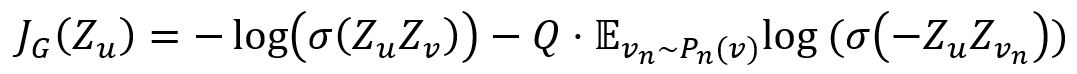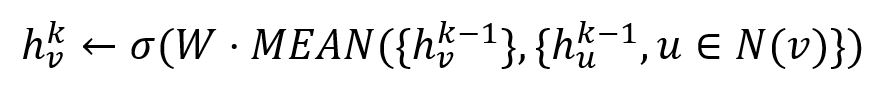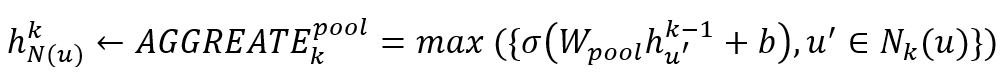# Change Nickname

Current Nickname:

Certifications

Certificate Issued at Valid until Serial No. File
Serial No. Valid until

Not having one? Apply now! >>>

Invoice

ProductName CreateTime ID Price File
ProductName CreateTime ID Price File

# GraphSAGE Train

## Overview

The model trained by GraphSAGE Train algorithm is to be used by GraphSAGE graph embedding algorithm. GraphSAGE was proposed by W.H Hamilton et al. of Stanford University in 2017.

Related material of the algorithm:

## Basic Concept

### GraphSAGE Train

GraphSAGE model parameters can be learned using standard stochastic gradient descent and backpropagation techniques.

The authors of GraphSAGE proposed the following graph-based loss function, adjusting weights according to it can encourage nearby nodes in the graph to have similar representations, while enforcing the representations of disparate nodes to be highly distinct:Node v is considered 'near' to node u if it occurs on the fixed-length random walk of node u, and it can be regarded as positive sample. Zu and Zv are output representations of nodes u and v, the larger their inner product, the more similar they are. `σ` is the sigmoid activation function. Q is the number of negative samples, vn denotes negative samples and Pn denotes a negative sampling distribution.

In cases where representations are to be used on a specific downstream task, the loss function can simply be replaced, or augmented, by a task-specific objective (e.g., cross-entropy loss).

### Aggregator Functions

Aggregator can convert a set of vectors into one vector. Unlike machine learning over N-dimensional lattices (e.g., sentences, or images), a node’s neighbors have no natural ordering. Thus, the aggregator function must operate over an unordered set of vectors, and ideally, it would be symmetric (i.e., invariant to permutations of its inputs) while still being trainable and maintaining high representational capacity. Ultipa's GraphSAGE Train algorithm supports two kinds of aggregators:

1. Mean Aggregator

Mean aggregator simply takes the elementwise mean of the vectors. When using this aggregator, the embedding generation algorithm of GraphSAGE directly calculate the the k-th representation of node:For example, there are 3 vectors [1,2], [4,3] and [3,4], they become vector [2.667,3] after being processed by the mean aggregator.

2. Pooling Aggregator

In pooling approach, each neighbor’s vector is independently fed through a fully-connected neural network; following this transformation, an elementwise max-pooling operation is applied to aggregate information across the neighbor set:where max denotes the element-wise max operator and `σ` is a nonlinear activation function.

## Command and Configuration

• Command：`algo(graph_sage_train)`
• Configurations for the parameter `params()`:
Name Type
Default
Specification
Description
dimension int 64 >0 Dimension of node embeddings as well as their hidden layer representations, i.e., number of node properties
node_property_names []`@<schema>?.<property>` / Node property, LTE needed Node property/properties to be used as features
edge_property_name `@<schema>?.<property>` / Numeric edge property, LTE needed Edge weight property/properties, schema can be either carried or not. During random walk, nodes only walk along edges with the specified properties and the probability of passing through these edges is proportional to the edge weight; if edge has multiple specified properties, the edge weight is the sum of these property values; the weight of all edges is 1 if not set
search_depth int 5 >0 Depth of random walk
sample_size []int [25, 10] Read from the model Length of the array is the maximum search depth K, elements in the array are the number of nodes sampled at layer-K, layer-K-1, ..., layer-1 successively
learning_rate float 0.1 [0, 1] Learning rate of each iteration
epochs int 10 >0 Number of times to traverse the graph, i.e., number of large cycles; before each epoch, neighborhood sampling will be re-done
max_iterations int 10 >0 Maximum number of iterations in each large cycle; each iteration uses the gradient of one randomly sampled batch
tolerance tolerance 1e-10 >0 The criterion for judging convergence at the end of each iteration, i.e., if the difference of the loss of the current iteration and the loss of the previous iteration is less than the tolerance, the algorithm stops
aggregator string mean mean or pool mean means to use mean aggregator, pool means to use pooling aggregator
batch_size int Number of nodes/threads >0 The number of nodes per batch; this is also used as the number of negative samples

## Algorithm Execution

#### 1. File Writeback

Configuration Data in Each Row Description File Type
model_name / The trained model JSON

Example: Run GraphSAGE Train algorithm, use properties age and hot as feature information, write the algorithm results back to file named model_1

``````algo(graph_sage_train).params({
node_property_names: ['age','hot']
}).write({
file:{
model_name: "model_1"
}
})
``````

#### 2. Property Writeback

Not supported by this algorithm.

#### 3. Statistics Writeback

This algorithm has no statistics.

### Direct Return

Not supported by this algorithm.

### Streaming Return

Not supported by this algorithm.

### Real-time Statistics

This algorithm has no statistics.